Question

# A spring with spring constant 141 N/m is used to pull a 336 N wooden block...

A spring with spring constant 141 N/m is used to pull a 336 N wooden block horizontally across a tabletop at constant velocity. The coefficient of kinetic friction between the block and the table is 0.33. By how much does this spring stretch from its equilibrium length (in meters)?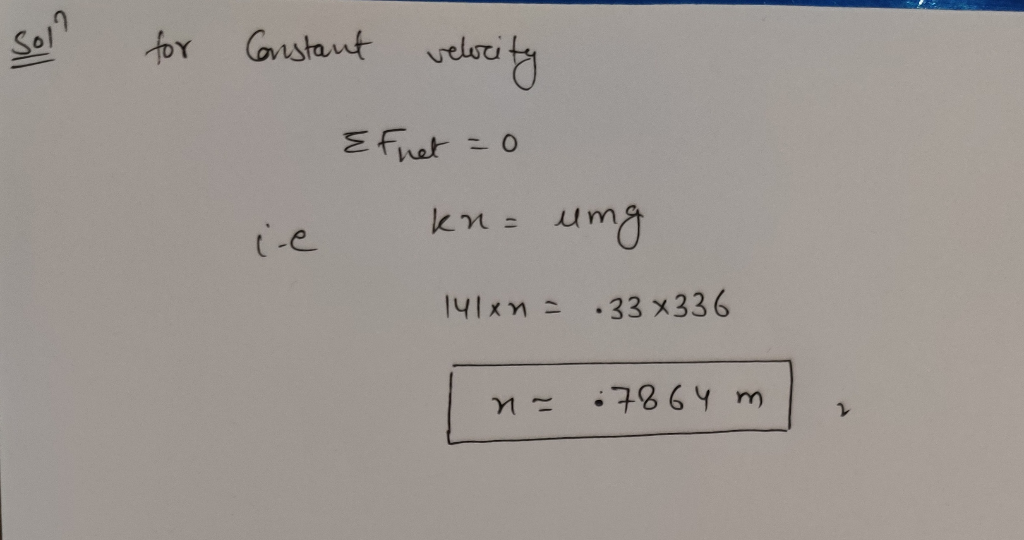hope this helps.

Please comment for any doubt or further clarification.

#### Earn Coins

Coins can be redeemed for fabulous gifts.

Similar Homework Help Questions
• ### 1) A 0.99 kg block of wood is attached to a spring whose spring constant, k...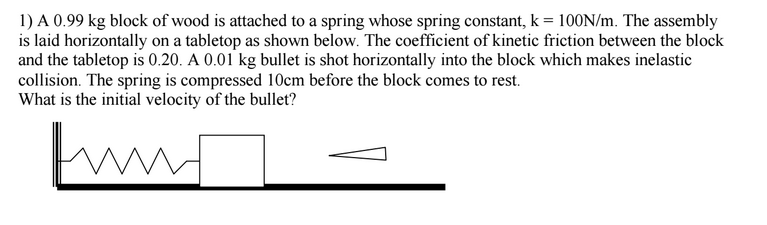1) A 0.99 kg block of wood is attached to a spring whose spring constant, k = 100N/m. The assembly is laid horizontally on a tabletop as shown below. The coefficient of kinetic friction between the block and the tabletop is 0.20. A 0.01 kg bullet is shot horizontally into the block which makes inelastic collision. The spring is compressed 10cm before the block comes to rest. What is the initial velocity of the bullet?

• ### wooden block with mass M 3 kg is lying on a horizontal table and is attached to a spring in its equilibrium position. It is hit by a bullet with mass m 5 g which moves horizontally. The bullet re...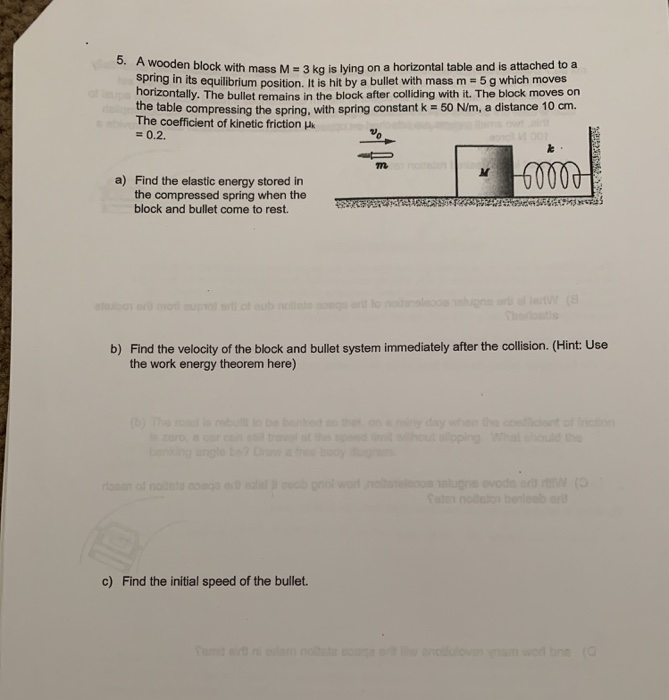wooden block with mass M 3 kg is lying on a horizontal table and is attached to a spring in its equilibrium position. It is hit by a bullet with mass m 5 g which moves horizontally. The bullet remains in the block after colliding with it. The block moves on the table compressing the spring, with spring constant k 50 Nm, a distance 10 cm. The coefficient of kinetic friction uk 0.2. a) Find the elastic energy stored in...

• ### Problem 5 A spring with a spring constant k=200 N/m is used as a launcher for...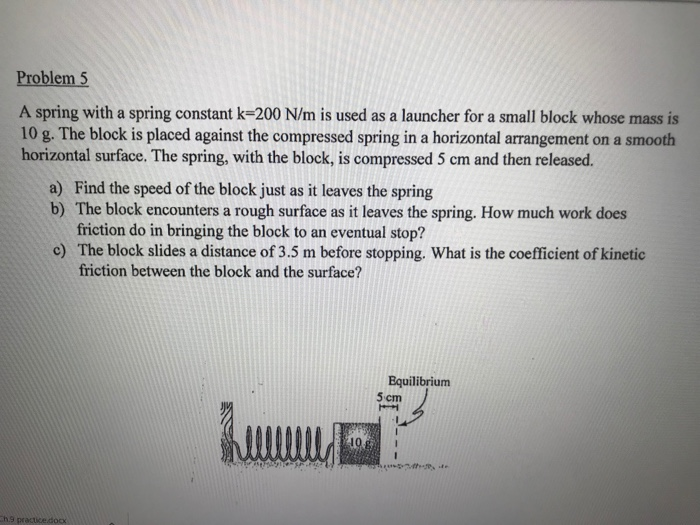Problem 5 A spring with a spring constant k=200 N/m is used as a launcher for a small block whose mass is 10 g. The block is placed against the compressed spring in a horizontal arrangement on a smooth horizontal surface. The spring, with the block, is compressed 5 cm and then released. a) Find the speed of the block just as it leaves the spring b) The block encounters a rough surface as it leaves the spring. How much...

• ### Problem 12: (10 points) PS 7-71: A spring with spring constant k is stretched a distance...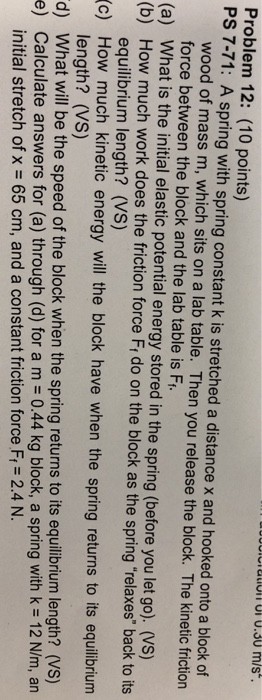Problem 12: (10 points) PS 7-71: A spring with spring constant k is stretched a distance x and hooked onto a block of wood of mass m, which sits on a lab table. Then you release the block. The kinetic friction force between the block and the lab table is F equilibrium length? (VS) length? (Vs) initial stretch of x 65 cm, and a constant friction force Fi 2.4 N. (a) What is the initial elastic potential energy stored in...

• ### A block of mass m=3.65kg is placed on a table and attached to a spring with...

A block of mass m=3.65kg is placed on a table and attached to a spring with spring constant k=150N/m. The spring is pulled so that the block slides over the table at a constant speed of 0.50 m/s. If the coefficient of kinetic friction between the block and the table is μ​k​​=0.3, how much is the spring extended beyond its equilibrium length? Give your answer in centimeters.

• ### A spring is used to fire a 100-g block horizontally. The spring has a spring constant...

A spring is used to fire a 100-g block horizontally. The spring has a spring constant of 20 N/m and is initially compressed by 5.0 cm. The block is initially at rest. a) What is the initial elastic potential energy? b) The spring is released. The block leaves the spring when the spring is at its relaxed state. What is the kinetic energy of the block as it leaves the spring? c) After the block leaves the spring, it enter...

• ### A 2kg block and an 8kg block are connected by a relaxed horizontal spring with spring-constant...

A 2kg block and an 8kg block are connected by a relaxed horizontal spring with spring-constant 300N/m. You pull the 8kg block with some constant horizontal force. There is a coefficient of kinetic friction between the blocks and the floor of μk = 0.3. After a time, the spring is stretched a certain constant amount, and the system moves with constant velocity. (a) Draw free-body diagrams for both blocks. (b) What pulling force did you apply? (c) How much is...

• ### 2. A block with mass m is forced against a horizontal spring of 100 N/m negligible...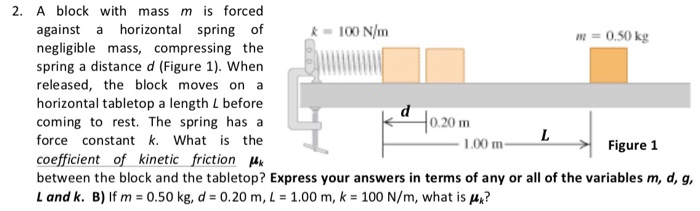2. A block with mass m is forced against a horizontal spring of 100 N/m negligible mass, compressing the spring a distance d (Figure 1). When released, the block moves on a horizontal tabletop a length L before coming to rest. The spring has a force constant k. What is the coefficient of kinetic friction between the block and the tabletop? Express your answers in terms of any or all of the variables m, d, g, L and k. B)...

• ### 3. A wooden block with mass 500g is pushed along a table to give it an...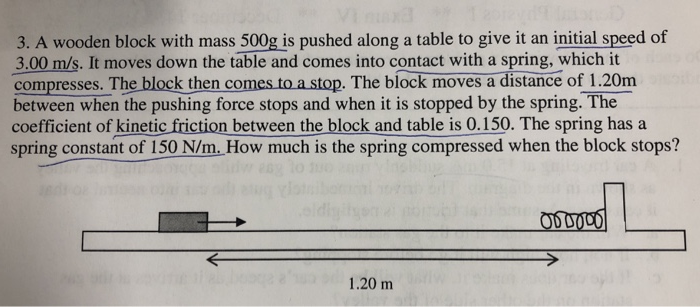3. A wooden block with mass 500g is pushed along a table to give it an initial speed of 3.00 m/s. It moves down the table and comes into contact with a spring, which it compresses. The block then comes to a stop. The block moves a distance of 1.20m between when the pushing force stops and when it is stopped by the spring. The coefficient of kinetic friction between the block and table is 0.150. The spring has a...

• ### A 0.458-kg box is placed in contact with a spring of stiffness 4.657×102 N/m. The spring...

A 0.458-kg box is placed in contact with a spring of stiffness 4.657×102 N/m. The spring is compressed 1.06×10-1 m from its unstrained length. The spring is then released, the block slides across a frictionless tabletop, and it flies through the air. The tabletop is a height of 1.400 m above the floor. What is the potential energy stored in the spring when it is compressed? What is the kinetic energy of the block just before it leaves the table...